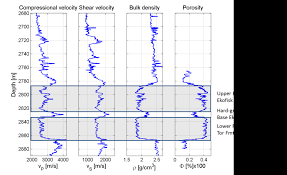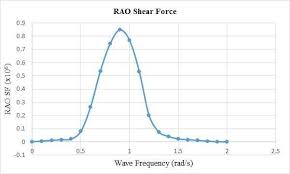## How to Calculate and Solve for Compressional or P Waves | Rock MechanicsThe image above represents compressional or P waves.

To compute for compressional or P waves, three essential parameters are needed and these parameters are Dynamic Bulk Modulus of Elasticity (kd), Dynamic Modulus of Rigidity (Gdand Elasticity of Rock Mass (p).

The formula for calculating compressional or P waves:

vp = √[kd + 4/3Gd] / p

Where:

vp = Compressional or P Waves
kd = Dynamic Bulk Modulus of Elasticity
Gd = Dynamic Modulus of Rigidity
p = Elasticity of the Rock Mass

Let’s solve an example;
Given that the dynamic bulk modulus of elasticity is 11, the dynamic modulus of rigidity is 30 and the elasticity of the rock mass is 23. Find the compressional or P waves?

This implies that;

kd = Dynamic Bulk Modulus of Elasticity = 11
Gd = Dynamic Modulus of Rigidity = 30
p = Elasticity of the Rock Mass = 23

vp = √[kd + 4/3Gd] / p
vp = √[11 + 4/3(30)] / 23
vp = √[11 + 40] / 23
vp = √ / 23
vp = 7.141 / 23
vp = 0.310

Therefore, the compressional or P waves is 0.310.

## How to Calculate and Solve for Shear Force or S Waves | Rock MechanicsThe image above represents shear force or S waves.

To compute for shear force or S waves, two essential parameters are needed and these parameters are Dynamic Modulus of Rigidity (Gd) and Elasticity of Rock Mass (p).

The formula for calculating shear force or S waves:

vs = √(Gd) / p

Where:

vs = Shear Force or S Waves
Gd = Dynamic Modulus of Rigidity
p = Elasticity of Rock Mass

Let’s solve an example;
Find the shear force or s waves when the dynamic modulus of rigidity is 12 and the elasticity of rock mass is 28.

This implies that;

Gd = Dynamic Modulus of Rigidity = 12
p = Elasticity of Rock Mass = 28

vs = √(Gd) / p
vs = √(12) / 28
vs = 3.46 / 28
vs = 0.12

Therefore, the shear force or S waves is 0.12.

Calculating the Dynamic Modulus of Rigidity when the Shear Force or S Waves and the Elasticity of Rock Mass is Given.

Gd = (vs x p)2

Where:

Gd = Dynamic Modulus of Rigidity
vs = Shear Force or S Waves
p = Elasticity of Rock Mass

Let’s solve an example;
Find the dynamic modulus of rigidity when the shear force or s waves is 21 and the elasticity of rock mass is 14.

This implies that;

vs = Shear Force or S Waves = 21
p = Elasticity of Rock Mass = 14

Gd = (vs x p)2
Gd = (21 x 14)2
Gd = (294)2
Gd = 86436

Therefore, the dynamic modulus of rigidity is 86436.